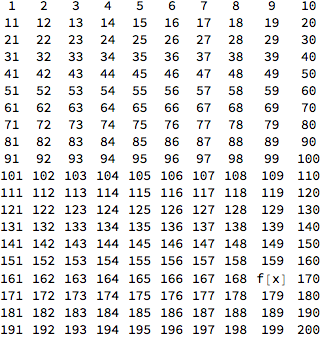## Errors

Obviously code doesn't always do what one expects it to. Often this is because the programmer or user is trying to do something wrong.

In many programming languages, the code will stop evaluating and the language will return an error. In Mathematica however, one of two things will happen:

### Errors as Messages

Particularly with built-in functions, when an error is encountered Mathematica will print an error message. For example, try evaluating the following

 Plot[Sin]

Plot::argr: Plot called with 1 argument; 2 arguments are expected.
 Plot[Sin]


You'll notice that Mathematica prints a small message telling us that Plot requires two arguments. Note however that what is returned is exactly what was input. This is because when Mathematica doesn't now how to evaluate a function, it simply returns the function and its arguments as is.

### Silent Errors

This behavior, returning expressions it can't evaluate, is critical to Mathematica programming, but can lead to some nasty bugs.

Consider the following:

 F[a_]:="~~Wow~~~"


Now say we want to format a big grid, but replace a random element with our the result of our function:

 thisCallsF[b_]:=
Grid@ReplacePart[Partition[Range,10],{RandomInteger, RandomInteger}->f[b]]
thisCallsF[x]Our grid isn't what we wanted it to be because we typed f instead of F and Mathematica saw no issue with our mistake. This is why it's critical to check your code to make sure you typed everything correctly--Mathematica won't do that for you.